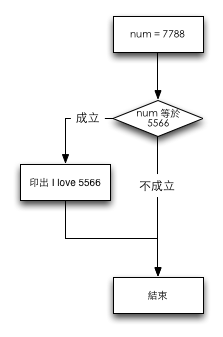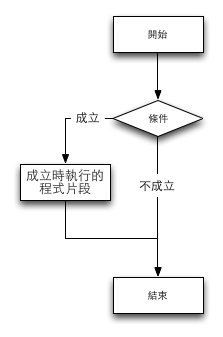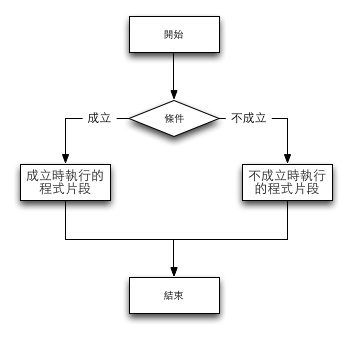# 向左走向右走 - 條件判斷式¶

```num = 5566
print 'The number is', num
if num == 5566:
print "I love 5566"
```

```The number is 5566
I love 5566```

`The number is 1234`

```The number is 5566
I love 5566```

```num = 7788
print 'The number is', num
if num == 5566:
print "I love 5566"
```

`The number is 7788``大客車走左邊，小客車走右邊`

`年齡大於等於18歲走左邊，否則走右邊`

```num == 5566
```

```if num = 5566:
print "I love 5566"```

## if 判斷式語法¶

```if 條件:
成立時執行的程式片段```## 程式區塊縮排¶

```num = 5566
if num == 5566:
print 'I love 5566'
print '5566 is the best'
print '5566 awesome'
```

```num = 5566
if num == 5566:
print 'I love 5566'
print '5566 is the best'
print '5566 awesome'```

```num = 5566
if num == 5566:
print 'I love 5566'
print '5566 is the best'
print '5566 awesome'
```

```print 'I love 5566'
```

## 判斷條件¶

```=>
=<```

```age = 20
if age >= 18:
print 'drink wine'
if age < 18:
print 'drink water'
```

`drink wine`

## if-else 判斷式¶

```age = 17
if age >= 18:
print 'drink wine'
else:
print 'drink water'
```

`drink water`

## if-else 判斷式語法¶

if-else 判斷式的語法本質上和 if 判斷式很相近，只是下面加了 else:

```if 條件:
成立時執行的程式片段
else:
不成立時執行的程式片段```## 邏輯運算式¶

`國文成積高於 50 分 且 數學成積高於 50 分`

`國文成積高於 50 分 或 數學成積高於 50 分`

```math = 50
chinese = 50
if math > 50 and chinese > 50:
print "You are qualified"
```

`You are qualified`

a and b
a or b

`國文成績不低於60分`

Python寫法:

```not chinese < 60
```

`數學成績大於60，或國文成績大於80且英文成績大於80`

Python寫法:

```math > 60 or (chinse > 80 and english > 80)
```